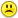+0

# logy x=3 logy (4x)=5

0
610
2

logy x=3 logy (4x)=5

Sep 25, 2014

#1
+5

logy x=3 logy (4x)=5

$$\small{\text{  \begin{array}{rclc|rclc} \log_y{ (x) } & = & 3 & | \quad y^{()} \quad & \quad \log_y {(4x)} & = & 5 \quad | \quad y^{()} \\ y^{\log_y{ (x) }} & = & y^3 & \quad & \quad y^{\log_y {(4x)}} & = &y^5 \\ x & = & y^3 & \quad & \quad 4x & = & y^5 \\ & & & & 4y^3 & = & y^5 \quad | \quad :y^3 \\ & & & & 4 & = & y^2 \quad | \quad \sqrt \\ & & & & 2 & = & y \\ x & = & 2^3 & & y & = & 2 \\ x & = & 8 & & y & = & 2 \\ \end{array}  }}$$

$$\small{\text{  \log_2{(8)}=3 \qquad \log_2{(4*8)}=5  }}$$.
Sep 26, 2014

#1
+5

logy x=3 logy (4x)=5

$$\small{\text{  \begin{array}{rclc|rclc} \log_y{ (x) } & = & 3 & | \quad y^{()} \quad & \quad \log_y {(4x)} & = & 5 \quad | \quad y^{()} \\ y^{\log_y{ (x) }} & = & y^3 & \quad & \quad y^{\log_y {(4x)}} & = &y^5 \\ x & = & y^3 & \quad & \quad 4x & = & y^5 \\ & & & & 4y^3 & = & y^5 \quad | \quad :y^3 \\ & & & & 4 & = & y^2 \quad | \quad \sqrt \\ & & & & 2 & = & y \\ x & = & 2^3 & & y & = & 2 \\ x & = & 8 & & y & = & 2 \\ \end{array}  }}$$

$$\small{\text{  \log_2{(8)}=3 \qquad \log_2{(4*8)}=5  }}$$heureka Sep 26, 2014
#2
+3

Nice one Heureka,I didn't even realise that I was being presented with 2 equations to solve simultaneously!I didn't understand WHAT the question was.  Perhaps you could present it a little better next time anonymous.

Heureka may not be around to interprete!Anyway, that is a really nice solution Heureka.Sep 26, 2014